## Area of Squares and Rectangles

Practice Unlimited Questions

#### What is the formula to calculate Area of a Rectangle and a Square?

Area of Rectangle  =  Length  ×  Breadth

Area of Square  =  Length  ×  Length

Let's find the area of the rectangle below.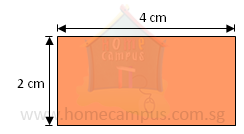To find the area of the rectangle, we find out how many one-centimetre squares we can fit into the rectangle.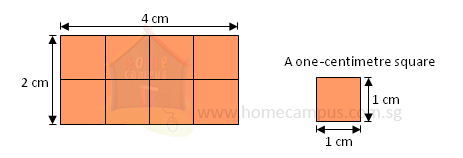Area of the rectangle  =  4  ×  2
=  8 cm2

We need 8 one-centimetre squares to make a rectangle 4 cm long and 2 cm wide.
The area of the rectangle is 8 cm2.

#### 1. What is the area of a rectangle of sides 3 cm by 2 cm?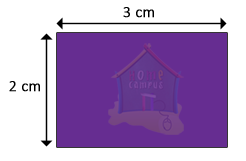To find out the area of the rectangle, we must find out how many 1-cm squares it is made up of.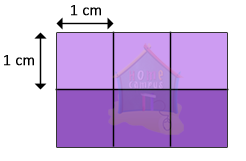A 3 cm by 2 cm rectangle is made up of 2 rows of 1-cm squares.
Each row has three 1-cm squares.
The rectangle is made up of six 1-cm squares.
So, the area of the rectangle is 6 cm2.

In other words, the area of the rectangle is the product of its length and breadth.

The longer side of a rectangle is called the length.
The shorter side is called the breadth.

Area of rectangle  =  Length  ×  Breadth

Area of the given rectangle
=  3  ×  2
=  6 cm2

#### 2. What is the area of a 4-cm square?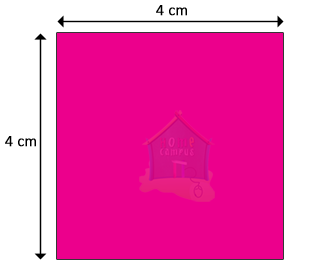To find out the area of the square, we must find out how many 1-cm squares it is made up of.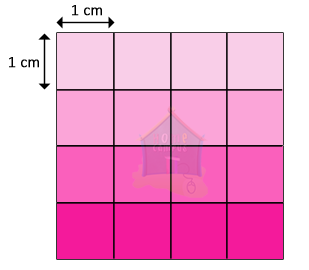A 4-cm square is made up of 4 rows of 1-cm squares.
Each row has four 1-cm squares.
The square is made up of sixteen 1-cm squares.
So, the area of the square is 16 cm2.

In other words, the area of the square is the product of its sides.

Area of square  =  Side  ×  Side

Area of the given square
=  4  ×  4
=  16 cm2

#### 3. Caroline wants to build a wall as shown below. What will be the area of the wall she builds?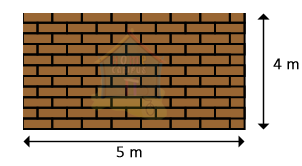Area of rectangle